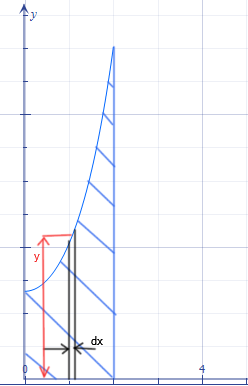# a) Calculate the volume of a revolution that occurs when the curve of y= e^x + e^{-x} rotates...

## Question:

a) Calculate the volume of a revolution that occurs when the curve of {eq}y= e^x + e^{-x} {/eq} rotates ones around the {eq}x {/eq}-axis in the interval {eq}0 \leq x \leq \ln 2. {/eq}

b) Calculate the volume generated by the rotation of the area decided by the coordinates and the curve {eq}y = e^x, x\in [0,1] {/eq}, around the line {eq}x = 1 {/eq}

## Solid of Revolution:

{eq}\\ {/eq}

An area bounded by a curve in the xy- plane, when rotated about an axis, generates a 3D shape.

The solid of revolution can be imagined as a composition of infinite elemental disks along the length of the curve.

The volume of such an elementary disk is simply: {eq}\mathrm{d}V = \pi r^2 \ \mathrm{d}t {/eq}

where, {eq}r {/eq} is the radius of the disk which is determined by the curve and the axis of rotation and {eq}\mathrm{d}t {/eq} is the thickness of the disk.

The volume is the solid of revolution is then obtained by summing (integrating) the volume of each disk: {eq}V = \int \mathrm{d}V {/eq}

{eq}\\ {/eq}

a)

The area under curve {eq}y=e^{x}+e^{-x}, \quad 0 \leq x \leq \ln{2} {/eq}; is sketched and shown in the figure below.Volume of the elementary disk of radius y and thickness {eq}\mathrm{d}x {/eq} (shown in figure) is: {eq}\mathrm{d}V = \pi r^2 \ \mathrm{d}x = \pi y^2 \ \mathrm{d}x {/eq}

The volume of the solid of revolution is obtained by integrating the volume of elementary disk:

{eq}\begin{align*} V &= \int_{x} \ \mathrm{d}V \\ &= \int_{0}^{\ln{2}} \pi y^2 \ \mathrm{d}x \\ &= \pi \int_{0}^{\ln{2}} (e^{x}+e^{-x})^2 \ \mathrm{d}x &&[\because y=e^{x}+e^{-x} ]\\ &= \pi \int_{0}^{\ln{2}} e^{2x}+e^{-2x} + 2e^{x}e^{-x} \ \mathrm{d}x \\ &= \pi \left[\frac{e^{2x}}{2} - \frac{e^{-2x}}{2} + 2x \right]_{0}^{\ln{2}} \\ \therefore \ \text{Volume of solid} &= \pi \left(2\ln \left(2\right)+\frac{15}{8}\right) \ \approx 10.2457 \end{align*} {/eq}

{eq}\\ {/eq}

b)

The area under curve {eq}y=e^{x}, \quad x \in [0,1] {/eq}; is sketched and shown in the figure below.As seen in the figure, we use two elementary disks each of thickness {eq}\mathrm{d}y {/eq}, one in the region {eq}1 \leq y \leq e {/eq} and other in the region {eq}0 \leq y \leq 1 {/eq}.

The radius of the disk in the region {eq}0 \leq y \leq 1 {/eq} is constant and equal to 1.

The curve as a function of y is given by: {eq}f(y) = x = \ln{y} {/eq}. The distance from the y- axis to the curve is {eq}x = \ln{y} {/eq}.

Therefore, the radius of the disk in the region {eq}1 \leq y \leq e {/eq} is: {eq}r = 1-x = 1-\ln{y} {/eq}

The volume of each elementary disk is obtained as:

{eq}0 \leq y \leq 1: \mathrm{d}V_1 = \pi r^2 \ \mathrm{d}y = \pi \ \mathrm{d}y {/eq}

{eq}1 \leq y \leq e: \mathrm{d}V_2 = \pi r^2 \ \mathrm{d}y = \pi (1-\ln{y})^2 \ \mathrm{d}y {/eq}

The volume of the solid of revolution therefore is:

{eq}\begin{align*} V &= \int_y \ \mathrm{d}V_1 + \int_y \ \mathrm{d}V_2 \\ &= \int_{0}^{1} \pi \ \mathrm{d}y + \int_{1}^{e} \pi (1-\ln{y})^2 \ \mathrm{d}y \\ &= \pi \Big[y\Big]_{0}^{1} + \pi \int_{1}^{e} 1-2\ln{y} + \ln^2{y} \ \mathrm{d}y \\ &= \pi + \pi\Big[y\Big]_{1}^{e} -2\pi\Big[y\ln{y}-y\Big]_{1}^{e} +\pi \Big[y\ln^2\left(y\right)-2\left(y\ln \left(y\right)-y\right)\Big]_{1}^{e} &&[\text{using standard results for: } \int \ln{x} \ \& \int \ln^2{x} ] \\ &= \pi + \pi(e-1-2+e-2) \\ \therefore \ \text{Volume of solid} &= \pi(2e-4) \ \approx 4.5131 \end{align*} {/eq}